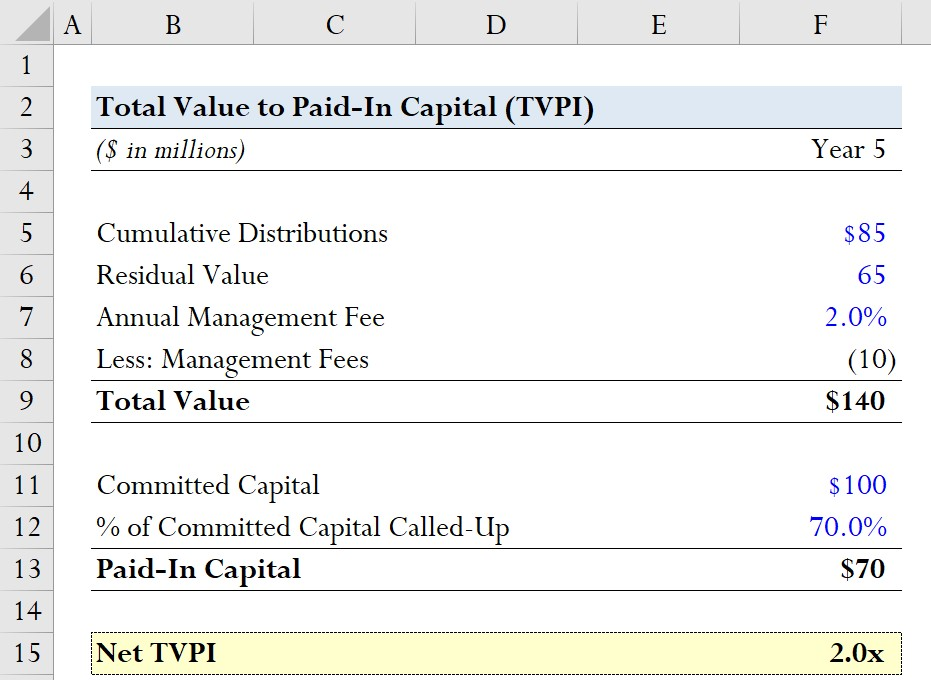# Total Value to Paid-In Capital (TVPI)

Guide to Understanding Total Value to Paid-In Capital (TVPI)## How to Calculate TVPI (Step-by-Step)

TVPI, shorthand for the “Total Value to Paid-In” capital multiple, is a metric used to measure the returns performance of a fund.

Formulaically, the TVPI multiple is the ratio between the total value of the fund’s realized distributions and unrealized holdings, compared to the paid-in capital from the limited partners (LPs).

• Total Value → The cumulative distributions to LPs (i.e. realized profits) and the residual value (i.e. unrealized potential profits)
• Paid-In Capital → The committed capital from LPs that have been “called” by the fund, i.e. paid-in by the LPs.

From the perspective of the investor, the TVPI answers, “How does the firm’s total realized and unrealized profits compare to the initial paid-in capital amount?”

Calculating the TVPI is relatively straightforward as it involves comparing the total value —i.e. realized profits and unrealized potential profits of the fund – relative to the capital contributed by the investor.

Therefore, to calculate TVPI, the total realized distributions to date and the estimated fair value of the remaining investments within the fund’s holdings are divided by the capital contributed to the fund to date.

• Cumulative Distributions → The total amount of capital returned to the LPs by the fund to date.
• Residual Value → The residual value is the estimated value of the fund’s current holdings and is often referred to as the net asset value (NAV).
• Paid-In Capital → The paid-in capital – i.e. the denominator in the TVPI multiple – represents the capital called and contributed by the LPs to the fund.

## Paid-In Capital vs. Committed Capital

While funds raise capital from LPs, the capital is not provided to the general partners (GPs) immediately.

The GPs must make a capital call to the LPs to request the committed capital.

Hence, the paid-in capital of an LP increases over the lifespan of the fund as the LPs contribute more of their committed capital.

The important takeaway here is that paid-in capital is NOT the same concept as committed capital.

## TVPI Formula

The formula for calculating the total value to paid-in capital multiple is as follows.

TVPI = (Cumulative Distributions + Residual Value) / Paid-In Capital

## Net vs. Gross TVPI: What is the Difference?

TVPI in most cases is a “net” measure, meaning management fees, carried interest (i.e. “carry”), and other expenses to LPs that reduce returns are taken into account.

Funds may occasionally report TVPI on a gross basis, but it is typically standard for the metric to be presented net of fees and expenses.

For instance, if an LP invested \$100k and the total value of realized and unrealized returns amounts to \$260k and there were \$10k in fees and carried interest, the net TVPI multiple would be 2.5x.

• TVPI = (\$260,000 – \$10,000) / (\$100,000) = 2.5x

## How to Interpret TVPI

The TVPI multiple is widely used to evaluate the performance of an investment fund by its limited partners (LPs).

As a general guideline, a TVPI equal to 1.0x means no profits – neither realized nor unrealized – were obtained in excess of fees.

• TVPI = 1.0x → Break-Even Profit
• TVPI > 1.0x → Positive Profit
• TVPI < 1.0x → Negative Profit

The main drawback to the TVPI is that the time value of money (TVM) is neglected, so it must be measured alongside the internal rate of return (IRR).

## TVPI Calculator – Excel Model Template

We’ll now move to a modeling exercise, which you can access by filling out the form below.Submitting ...

## TVPI Multiple Calculation Example

Suppose there is a private equity fund with a total of \$100 million in committed capital from their LPs.

Of the \$100 million, 70% of the committed capital has been called as of Year 5, so the paid-in capital is \$70 million.

• Committed Capital = \$100 million
• % of Committed Capital Called = 70%
• Paid-In Capital = 70% * \$100 million = \$70 million

Calculating the numerator will consist of adding together the cumulative distributions and the residual value, which we’ll assume to be \$85 million and \$65 million, respectively.

• Cumulative Distributions = \$85 million
• Residual Value = \$65 million

Since the net TVPI is being calculated, we must also deduct any management fees accumulated to date.

We’ll assume the annual management fees are 2.0% of the total commitment capital, so the management fees equal \$10 million.

• Management Fees = (2.0% * \$100 million) * 5 Years = \$10 million

The total value of the fund as of Year 5 is \$140 million.

• Total Value = \$85 million + \$65 million – \$10 million = \$140 million

Since the fund returns are not fully realized and only \$85 million was distributed relative to the \$100 million in committed capital – i.e. there is still uncalled capital and “unrealized” residual value – the GPs have not received any carried interest yet.

GPs earn carry only after the LPs have been distributed the entirety of their initial capital contribution (i.e. the return of their original capital commitment) and then the LPs receive 100% of the proceeds until the preferred return (or “hurdle rate”) is met.

The preferred return in private equity is typically 8.0% and once the minimum threshold is met, the GP “catch-up” clause is triggered with the traditional 80/20 distribution split applied to proceeds thereafter.

Upon dividing the total value of \$140 million by the \$70 million in paid-in capital, we arrive at a net TVPI of 2.0x as of Year 5.

• Net TVPI = \$140 million / \$70 million = 2.0xMaster LBO Modeling Our Advanced LBO Modeling course will teach you how to build a comprehensive LBO model and give you the confidence to ace the finance interview.